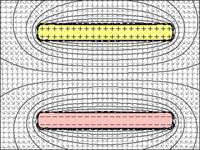Search

# Topic 5.1 Electric fields

Updated: Oct 22, 2019

In this post we are going to talk about electric fields. What is an electric field? How does an electric field work?

In order to understand the concept of electric fields we have to deal with electric charge. Electric charge is a fundamental property of some subatomic particles. A particle or object can be either positively or negatively charged. Two subatomic particles are protons and electrons. Electrons are negatively charged and protons are positively charged. Electric forces exist between all charged particles or objects. Opposite charges attract, like charges repel. The total charge in any isolated system is constant according to the law of conservation of charge. Charge is measured in the unit coulomb, C. One coulomb is the charge that flows past a point in one second if the current is one amp. Charge is given the symbol q.An electric field is a region of space in which any charge would experience an electric force. Its unit is electric field strength, E. In an electric field particles or objects don’t have any contact with each other. Electric field strength is at a point is defined as a force per unit charge (1C) that would be experienced by a small positive test charge placed at that point. Its equation is E=F/q where F is force and q is charge. Its units are N C ^-1. Electric field strength is a vector quantity. If two or more fields exist at the same place then the resultant field strength can be determined by vector addition.

An electric field can be shown through by field lines. Electric field lines shows the direction of the force that would be exerted on a positive test charge if it was placed at that point from positive to negative. A field is strongest where the lines are the closest as seen in Figure 2.In this post I have introduced you to electricity. I will continue with this topic in my upcoming blog posts.

Thanks for reading and stay tuned for more!

Sources:

Physics for the IB diploma - John Allum, Christopher Talbot

Figure 1: https://www.quora.com/Why-do-the-same-charges-repel-each-other

Figure 2: http://www.excelatphysics.com/electric-field.html